# PHYS 1444 Section 002 Lecture 10 Monday Sept

• Slides: 14
Download presentationPHYS 1444 – Section 002 Lecture 10 Monday, Sept. 30, 2019 Dr. Jaehoon Yu CH 23 – Electrostatic Potential Energy • Chapter 24 Capacitance etc. . – – Capacitors in Series or Parallel Electric Energy Storage Effect of Dielectric Today’s homework is homework #7, due 11 pm, Wednesday, OAnnouncements • Bring out Special Project #3 • Mark your calendar for the two triple extra credit colloquiua – Oct. 29: Professor Liangtao Wang of U. of Chicago – Nov. 13: Professor Hitoshi Murayama of UC Berkeley • Quiz #2 – This Wednesday, Oct. 2 at the beginning of the class – Covers CH 22. 1 through what we cover in class today (CH 24. 3 or 24. 4) – Bring your calculator but DO NOT input formula into it! • Cell phones or any types of computers cannot replace a calculator! – BYOF: You may bring a one 8. 5 x 11. 5 sheet (front and back) of handwritten formulae and values of constants for the quiz – No derivations, word definitions or solutions of any problems! – No additional formulae or values of constants will be provided! Monday, Sept. 30, 2019 PHYS 1444 -002, Fall 2019 Dr. Jaehoon Yu 2Electrostatic Potential Energy • Consider a case in which a point charge q is moved between points a and b where the electrostatic potential due to other charges in the system is Va and Vb • The change in electrostatic potential energy of q in the field by other charges is • Now what is the electrostatic potential energy of a system of charges? – Let’s choose V=0 at r=∞ – If there are no other charges around, single point charge Q 1 in isolation has no potential energy and is under no electric. PHYS force Monday, Sept. 30, 1444 -002, Fall 2019 3 2019 Dr. Jaehoon Yu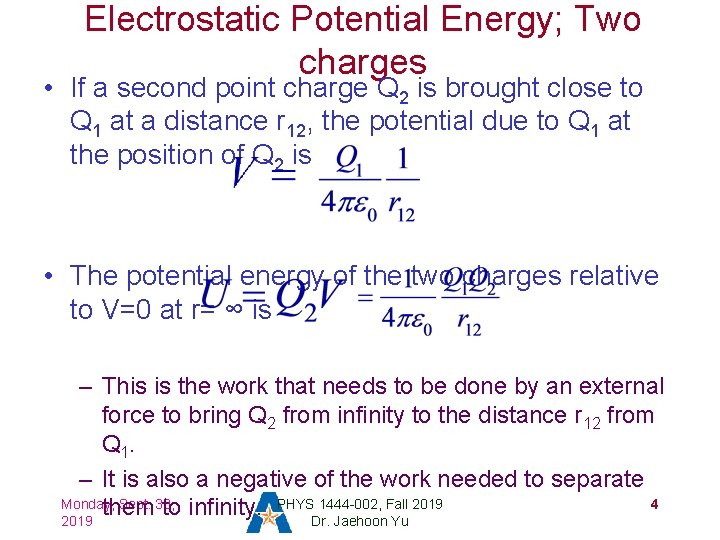Electrostatic Potential Energy; Two charges • If a second point charge Q 2 is brought close to Q 1 at a distance r 12, the potential due to Q 1 at the position of Q 2 is • The potential energy of the two charges relative to V=0 at r= ∞ is – This is the work that needs to be done by an external force to bring Q 2 from infinity to the distance r 12 from Q 1. – It is also a negative of the work needed to separate Monday, Sept. 30, PHYS 1444 -002, Fall 2019 4 them to infinity. 2019 Dr. Jaehoon Yu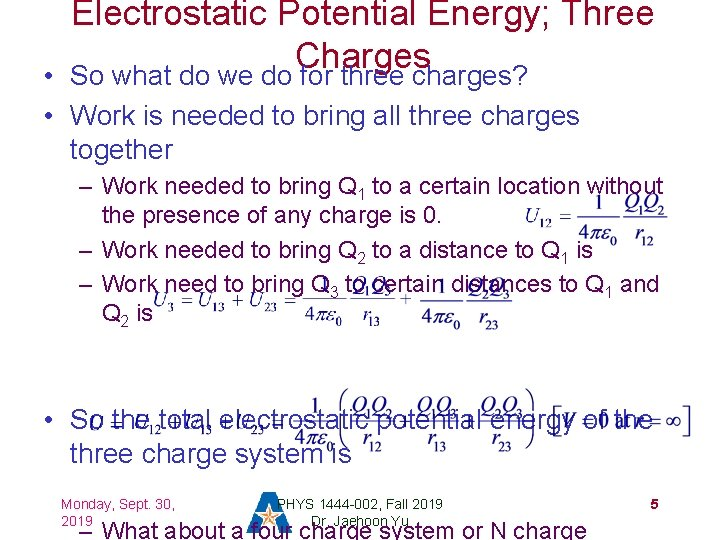Electrostatic Potential Energy; Three Charges So what do we do for three charges? • • Work is needed to bring all three charges together – Work needed to bring Q 1 to a certain location without the presence of any charge is 0. – Work needed to bring Q 2 to a distance to Q 1 is – Work need to bring Q 3 to certain distances to Q 1 and Q 2 is • So the total electrostatic potential energy of the three charge system is Monday, Sept. 30, 2019 PHYS 1444 -002, Fall 2019 Dr. Jaehoon Yu – What about a four charge system or N charge 5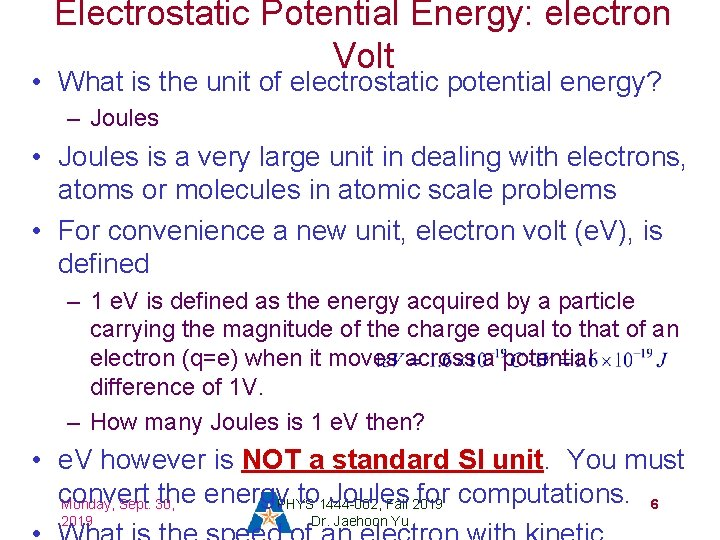Electrostatic Potential Energy: electron Volt • What is the unit of electrostatic potential energy? – Joules • Joules is a very large unit in dealing with electrons, atoms or molecules in atomic scale problems • For convenience a new unit, electron volt (e. V), is defined – 1 e. V is defined as the energy acquired by a particle carrying the magnitude of the charge equal to that of an electron (q=e) when it moves across a potential difference of 1 V. – How many Joules is 1 e. V then? • e. V however is NOT a standard SI unit. You must convert the energy. PHYS to 1444 -002, Joules for computations. 6 Monday, Sept. 30, Fall 2019 Dr. Jaehoon Yu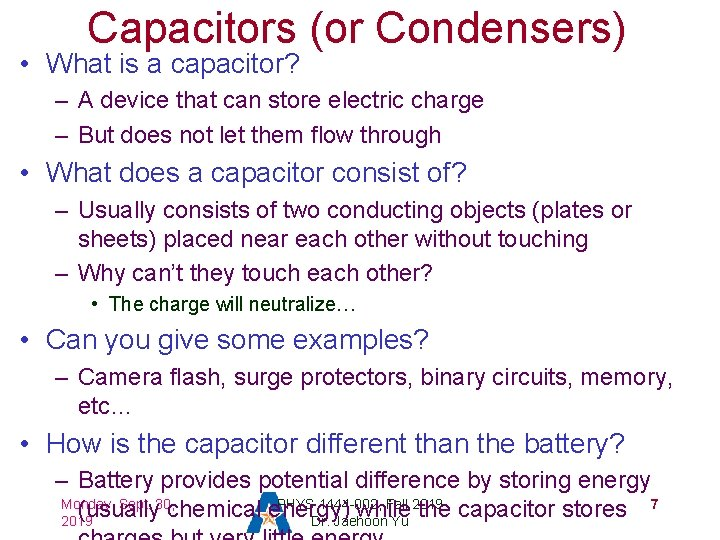Capacitors (or Condensers) • What is a capacitor? – A device that can store electric charge – But does not let them flow through • What does a capacitor consist of? – Usually consists of two conducting objects (plates or sheets) placed near each other without touching – Why can’t they touch each other? • The charge will neutralize… • Can you give some examples? – Camera flash, surge protectors, binary circuits, memory, etc… • How is the capacitor different than the battery? – Battery provides potential difference by storing energy Monday, Sept. 30, PHYS 1444 -002, Fall 2019 7 (usually chemical energy) while the capacitor stores 2019 Dr. Jaehoon Yu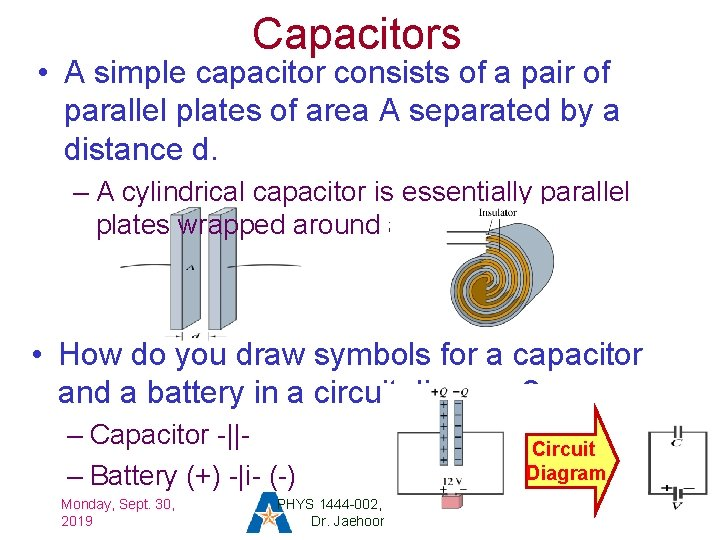Capacitors • A simple capacitor consists of a pair of parallel plates of area A separated by a distance d. – A cylindrical capacitor is essentially parallel plates wrapped around as a cylinder. • How do you draw symbols for a capacitor and a battery in a circuit diagram? – Capacitor -||– Battery (+) -|i- (-) Monday, Sept. 30, 2019 PHYS 1444 -002, Fall 2019 Dr. Jaehoon Yu Circuit Diagram 8Capacitors • What do you think will happen if a battery is connected ( or the voltage is applied) to a capacitor? – The capacitor gets charged quickly, one plate positive and the other negative in equal amount. • The battery terminals, the wires and the plates are conductors. What does this mean? – All conductors are at the same potential. And? – So the full battery voltage is applied across the capacitor plates. • So for a given capacitor, the amount of charge stored on each plate issoproportional to. Q or V. C iscapacitor the property of a capacitor does not depend on the potential difference Vba between the plates. Monday, Sept. 30, PHYS 1444 -002, Fall 2019 9 How would you write this formula? C/V Dr. or. Jaehoon Farad (F) Normally use μF or p. F 2019 YuDetermination of Capacitance • C can be determined analytically for a capacitor with a simple geometry and air in between. • Let’s consider a parallel plate capacitor. – Plates have area A each and separated by d. • d is smaller than the length, and so E is uniform. – E for parallel plates is E=σ/ε 0, σ=Q/A is the surface charge density. • E and V are related • Since we take the integral from the lower potential (a) to the higher potential (b) along the field line, we obtain C only depends on the area and the distance of • the plates and the permittivity of the • Monday, So from Sept. 30, the formula: PHYS 1444 -002, Fall 2019 10 medium between them. 2019 Dr. Jaehoon Yu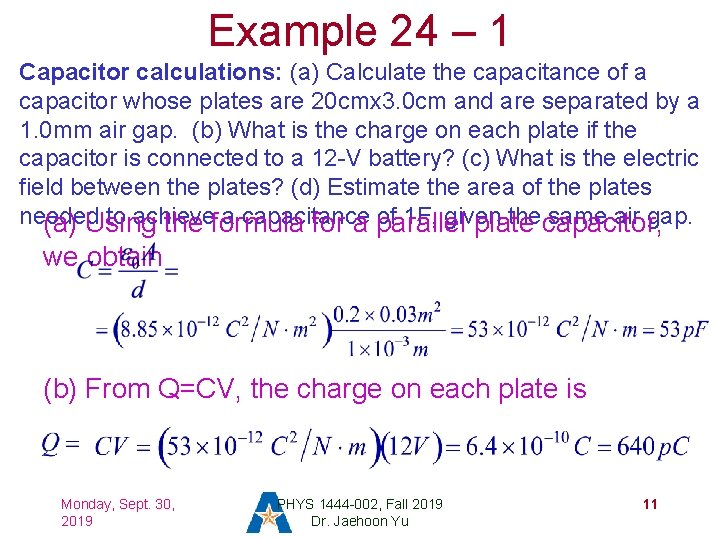Example 24 – 1 Capacitor calculations: (a) Calculate the capacitance of a capacitor whose plates are 20 cmx 3. 0 cm and are separated by a 1. 0 mm air gap. (b) What is the charge on each plate if the capacitor is connected to a 12 -V battery? (c) What is the electric field between the plates? (d) Estimate the area of the plates needed to achieve a capacitance of 1 F, given thecapacitor, same air gap. (a) Using the formula for a parallel plate we obtain (b) From Q=CV, the charge on each plate is Monday, Sept. 30, 2019 PHYS 1444 -002, Fall 2019 Dr. Jaehoon Yu 11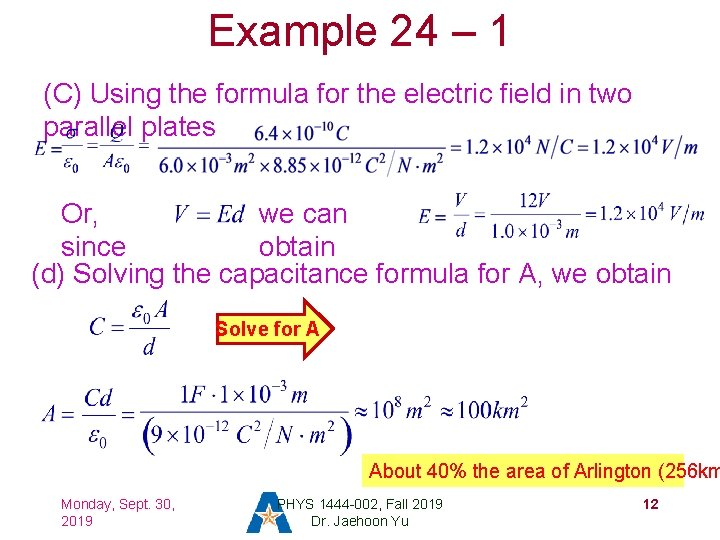Example 24 – 1 (C) Using the formula for the electric field in two parallel plates Or, we can since obtain (d) Solving the capacitance formula for A, we obtain Solve for A About 40% the area of Arlington (256 km Monday, Sept. 30, 2019 PHYS 1444 -002, Fall 2019 Dr. Jaehoon Yu 12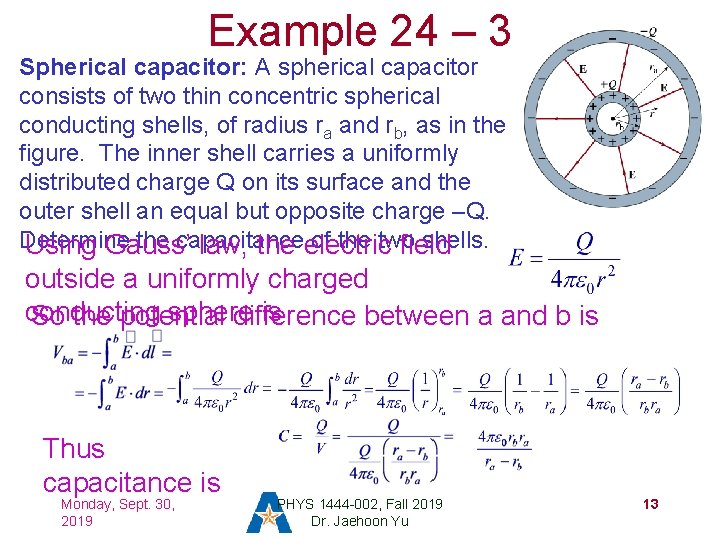Example 24 – 3 Spherical capacitor: A spherical capacitor consists of two thin concentric spherical conducting shells, of radius ra and rb, as in the figure. The inner shell carries a uniformly distributed charge Q on its surface and the outer shell an equal but opposite charge –Q. Determine the capacitance of the two shells. Using Gauss’ law, the electric field outside a uniformly charged conducting sphere is So the potential difference between a and b is Thus capacitance is Monday, Sept. 30, 2019 PHYS 1444 -002, Fall 2019 Dr. Jaehoon Yu 13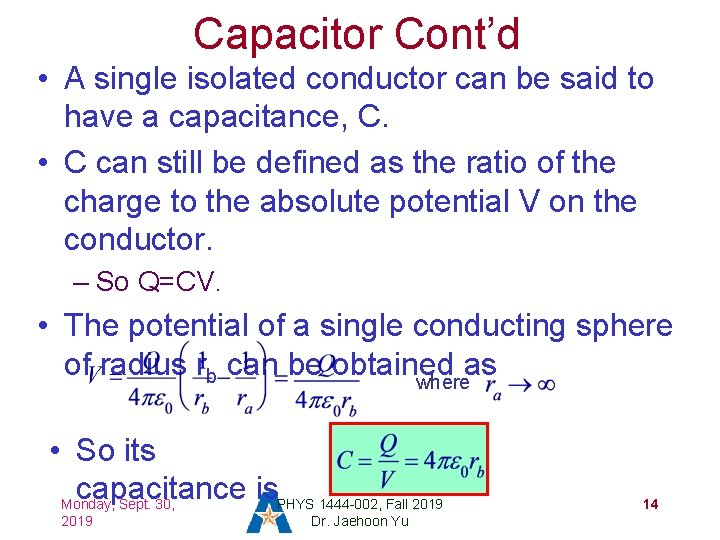Capacitor Cont’d • A single isolated conductor can be said to have a capacitance, C. • C can still be defined as the ratio of the charge to the absolute potential V on the conductor. – So Q=CV. • The potential of a single conducting sphere of radius rb can be obtained as where • So its capacitance is. PHYS 1444 -002, Fall 2019 Monday, Sept. 30, 2019 Dr. Jaehoon Yu 14